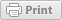KNOWLEDGE BASE
Everything you need to know about using the Kinduct Platform
Follow

# Create a Calculated MetricThe Calculation Builder functionality allows you to run calculated formulas to create new metrics within the platform. This tool allows you to create calculations using metrics from any source of data that is brought into the platform. This includes the ability to complete basic operations such as add​, subtract​, multiply​ and divide​ metrics, as well as functions like average​, max​, age​, previous​ and days since​. When a new metric is created from a calculation, it can then be used throughout the site like any other metric.

A benefit of the calculation builder functionality is the ability to utilize other existing metrics in the platform within the builder. For example, a metric combining workout minutes​, mood​, and Rate of Perceived Exertion​ (RPE).

To create a calculated metric:

1. Click the Admin tab, click Data Management and then select Metrics.The Metrics Management screen opens:2. Click Create a Calculated Metric.3. From the Create a Calculated Metric screen, enter a Metric Label. This is the name of the Metric that will appear in Reporting​ and Metrics Management​.
NOTE: Do not use the following reserved names as a label: abs, age, average, avg, camp, cube, date, days_since, diff, league, max, min, name, number, position, pow, previous, rand, rolling, round, segment, square, sum.
4. Enter a Metric Source.​ The metric source allows you to organize your metrics into groups of related metrics. Examples are Journals, RPE, or any custom source name.5. Select desired Metric Calculation Options:
Require All Values: Selecting this option requires all metrics in the formula to have numeric values. This ensures that a valid calculation can occur. If this option is not selected, a calculation will still occur even if one or more metrics in the formula do not have numeric values.
Perform calculation using only metrics recorded on the same date: Selecting this option will calculate using metric values that are recorded on the same date.
Perform calculation using the latest possible metric values: Selecting this option will calculate using the latest available metric values.
6. Click in the Calculation field to enter operator, function and/or metrics for your calculation. Once you click in the field, a list displays to select from possible operators, available functions and available metrics.
NOTE:  Suggested operators only display when they can be used contextually. As you begin typing functions or metric names, relevant options automatically display to select from. You will see a warning if a metric or function is invalid.7. Click the arrows to expand and locate possible options, or start typing to select from suggested list.
8. Once your formula is complete and is indicated as valid, click Save. Example of a validated formula, sum of 5 journal metrics for a Total Wellness Score:9. Once the new Calculated Metric is saved, you can find it in the Metrics Management screen where you can edit, delete or copy the calculated metric. The Metrics Management screen is where you can also enable SCALING, edit the PRECISION value (range -3 to 5), manage ACCESS and choose to show or hide the metric.## Available Functions

There are 15 functions available. Hover over the function name to display a description and example of the function.

 Function Description ABS Returns the absolute value of a number, a number without its sign. AGE Returns the age of the user based on the birth date listed in profile. AVERAGE Returns average of a list of numbers, the sum of a list of numbers divided by the number of numbers in the list. CUBE Returns the cube of a number, the result of the number multiplied by itself three times. DAYS_SINCE Returns the number of days between the specified data and today. e.g. DAYS_SINCE(2014-04-20). DIFF Returns the differential, a change in the linearization of a function. MAX Returns the largest value in a series of values. MIN Returns the smallest value in a series of values. POW Returns the results of a number raised to a power. PREVIOUS Returns the previous value of the specific metric going back by either occurrence count or days previous to today. Count is the the default represented by 0. To specify days change the 3rd parameter to 1. RAND Returns a random number between start​ and end​ (inclusive). ROLLING Returns the rolling average of the metric over specified number of days. To exclude days where no data is captured change the 3rd parameter to 1. ROUND Returns a number rounded to a specified number of digits. SQUARE Returns the square of a number, the result of the number multiplied by itself. SUM Returns the sum of a list of numbers, the result of adding all numbers in a list together.

## Calculation Examples

### Acute Chronic Ratio### Percent Change of a Metric